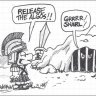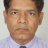# Combining best of both setups (chart + watch list)##### Member
Charts are helpful in capturing trend over time. Watch lists are helpful in capturing snapshots of multiple symbols. Can we combine these attributes in one chart? For example, daily 5/13 ema crossover may signal down/up trend for a particular stock and it can be plotted as a red / green green dot. We can plot ten symbols like ( MSFT, AAPL, AMD, TSLA, SPY, QQQ, MU, NVDA, NFLX, FB) in a chart. A major sell off or bouncing back or recovery after pull back can be easily spotted in the chart. This may be called dashboard. Can anyone help in this development of thinkscript. We can explore different criteria for red dots or green dots.

T

#### tomsk

##### Well-known member
VIP
@samiranadhikari You can either plot all these 10 symbols on a chart which in my opinion would be a bit cluttered, or alternatively you may wish to use labels, color coded to denote a 5/13 EMA crossover/crossdown as the case may be.

•##### Member
The idea was to create a portfolio and watching symbols over time. One can add or rebalance positions.

T

#### tomsk

##### Well-known member
VIP
@samiranadhikari Ah, that's different then. You can absolutely create your own portfolio/index that you'd like to watch. Here's one such version from Mobius that I have in my files, feel free to play with this as needed. Read the comments in the code. Since this was posted 3 years ago, you may wish to revise the weightings. It's a good template to base any future work from

Code:
``````# My Index
# Mobius
# 7.19.2016

# Here is an index I use. You will need to decided on how you want you symbols weighted.

input Plot_Close = yes;
input MA_Type = AverageType.Hull;
input Plot_MA = yes;
input MA_Length = 8;

script Scale {
input c = close;
input Min = 0;
input Max = 0;
def hh = HighestAll(c);
def ll = LowestAll(c);
plot Range = (((Max - Min) * (c - ll)) /  (hh - ll)) + Min;
}

def cC = ((close("GOOGL") *  .276) +
(close("BRK/B") *  .986) +
(close("AAPL")  * 2.203) +
(close("FB")    * 1.150) +
(close("XOM")   * 1.670) +
(close("JNJ")   * 1.109) +
(close("MSFT")  * 3.162) +
(close("PG")    * 1.071) +
(close("WFC")   * 2.042) +
(close("CHL")   * 1.647) +
(close("JPM")   * 1.471) +
(close("NVS")   *  .957) +
(close("WMT")   * 1.265) +
(close("PTR")   *  .736) +
(close("GE")    * 3.753));
def min = LowestAll(close);
def max = HighestAll(close);
plot c = Scale(c = cC, min = min, max = max);
c.SetHiding(!Plot_Close);
c.AssignValueColor(if c >= c then color.cyan else color.yellow);
plot MA = if Plot_MA
then MovingAverage(AverageType = MA_Type, c, Ma_Length)
else double.nan;
MA.AssignValueColor(if MA >= MA then color.cyan else color.yellow);
# End Code``````

•#### markos

##### Well-known member
VIP
@tomsk shouldn't Input Max = 100 vs 0 that our friend has?

T

#### tomsk

##### Well-known member
VIP
@markos Nope, those are local variables used within the script() function used as a placeholder. and are overwritten when the actual variables are passed from the actual function call. Besides Mobius doesn't make this sort of mistakes!

To help you better understand this, I have written a simple script to illustrate this. The result that is plotted is 8000 as we'd expect. Good question though. I literally had the same question when I first dabbled in the script() function years ago.

Code:
``````def x = 2000;
def y = 8000;

declare lower;

script MaxMin {
input min = 10;
input max = 20;

plot result = Max(min,max);
}

plot c = MaxMin(min = x, max = y);
# End Code``````

Last edited:
•markosExploring Scan Combining Unusual volume and near 52 weeks high ... Playground 26